# Excel Substring formula

There is no specific formula in Excel to extract a substring from the other string. A substring is a string that is extracted from a complete string. Excel does not provide any direct function for this. But it offers several others functions, such as LEFT, RIGHT, MID, TRIM, LEN, FIND, SUBSTITUTE, REPT, and MAX. They help the users to extract the substrings in different ways.

Some of these functions are used directly and some are used inside another function to get the targeted substring.

In this chapter, we will discuss the methods to find the substring with their examples. Then, you can use them accordingly with your data. You will find these functions very simple and easy to use.

Topics covered in this chapter:

These methods, we will discuss in this chapter with detailed explanation.

## LEFT()

The Left() function extracts the characters from the left side of the string. The user needs to provide the string cell reference (or string itself in double-quotes) and the number of characters to be extracted from it.

Syntax

Look at an example below:

Example

We have a string in B4 cell on which we will apply the left() function to extract the five leftmost characters from it. Write the formula as given below:

=LEFT(B4, 5)Hit the Enter key and see that five characters have been successfully subtracted from the left and we got a new substring Kavya from Kavyansh.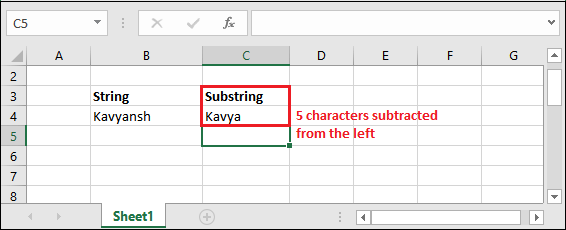#### Note: The string can contain text, numbers, and special characters as well. It can work on all types of strings.

The right() function helps to extract the characters from the right of the original string. This function takes two argument: one is text string (string cell reference) and another is number of characters to be extracted from the right.

Syntax

Syntax is almost the same as the Left() function of Excel. Just name of the function is different.

Look at an example below:

Example

We have a string in B4 cell on which we will apply the right() formula to extract the four rightmost characters from it. Write the formula as given below:

=RIGHT(B4, 4)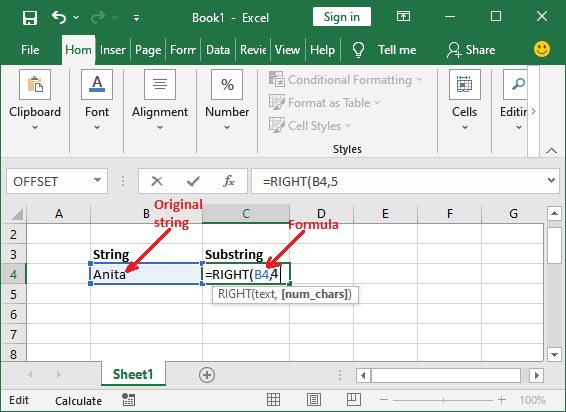Hit the Enter key and see that four characters have been successfully subtracted from the right and we got a new substring Nita from Anita.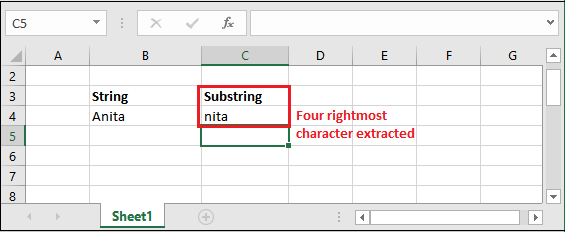## MID()

Now, this one is a bit different function. It extracts the substring neither from left nor from right. It extracts a substring from the middle of the string.

This function takes three arguments, i.e., text string, position to start extraction, and the number of characters to be extracted from the string.

Syntax

Look at an example below:

Example

We have a string in B4 cell on which we will apply the MID() formula to extract the substring from the middle of the string. Write the formula as given below:

=MID(B4, 2,4)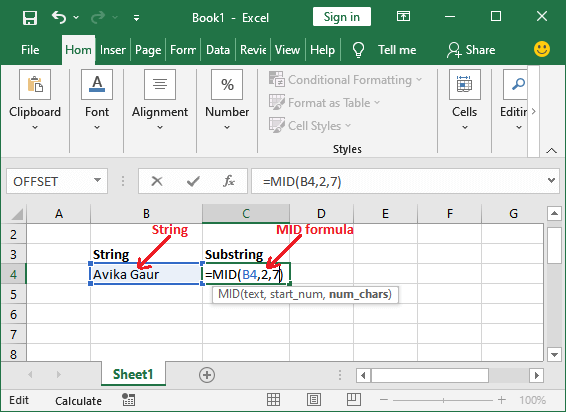See the substring extracted from the string Avika Gaur, and the returned string is Vika Ga.## TRIM()

TRIM() function removes the leading and trailing spaces from the string and returns the remaining extracted substring. This function takes only one argument, i.e., text string (cell reference of string).

Syntax

Look at an example below:

Example

We have a string ( Hello World!) in B4 cell on which we will apply the TRIM() formula to remove the leading and trailing spaces and get the extracted substring.

For this, write the formula as showing below:

=TRIM(B4)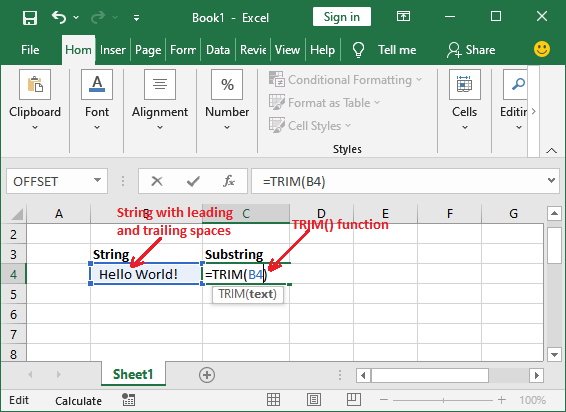See the substring extracted from the string Hello world! that was initially containing spaces in beginning and end.For detailed learning of TRIM() function, click here (https://www.tutoraspire.com/remove-spaces-in-excel#:~:text=Remove%20spaces%20using%20TRIM()%20function,-Extra%20space%20in&text=TRIM()%20function%20is%20a,between%20space%3B%20it%20removes%20all.) and follow our complete tutorial with example explanation.

## Extract a substring before a specific character

Sometimes we need a string to be extracted before a specific character. This may usually be required while extracting the user name from the email id. For example, tutoraspire2011 (user name) from [email protected] (email id).

This method will help you to achieve this type of result. It requires two functions: LEFT() and SEARCH().

They are used together, where SEARCH() is used inside the LEFT() function as the second parameter. So, the result returned by the SEARCH() function is an integer number that is used in place of number of characters.

Syntax

The following is the syntax to extract the substring before a specific character.

Here,

• textString will contain the string from which the substring will be extracted.
• Char parameter takes the character before which a substring will extract.

### Example,

We have an email id [email protected] in B4 cell. Now, extract a substring (user name) available before the @ character from it, use the following formula:

=LEFT(B4, SEARCH(“@”, B4) -1)Hit the Enter key and get the extracted user name (substring before @ symbol) from the email id.### How this formula worked?

=LEFT(B4, SEARCH(“@”, B4) -1) for the string [email protected].

The first thing to know that – this formula will execute from “right to left”. It requires only a few simple statements to understand how it works. Read them carefully.

1. Firstly, the SEARCH() function will return the position of @ symbol in the original string, i.e., 11 here.
2. Now, 1 will be subtracted from the position value (11) returned by the SEARCH() function. For example, 11-1 = 10 to exclude the @ symbol.
3. This resultant value (10) will be used as the second parameter inside the LFTT() function at the place of a number of characters to be extracted.

In the end, the LEFT() function will execute and return the 10 leftmost characters from the original string, i.e., tutoraspire.

## Extract a substring after a specific character

Similar to the above method, you can also extract the substring after a specific character. For example, you can extract the domain name from the string after a particular character, i.e., microsoft.com (domain), from [email protected] (email id). This method will help you to achieve this result.

It requires three functions: RIGHT(), LEN(), and SEARCH() function. Here, LEN() and SEARCH() are used inside the RIGHT() function, whose returned value is used as the second parameter. So, the resultant value returned by them will work as the number of characters to be extracted.

Syntax

The following is the syntax to extract the substring after a specific character.

Here,

• textString will contain the string from which the substring will be extracted.
• The char parameter takes that specific character after which a substring will extract.

### Example,

We have an email id [email protected] in B4 cell. Now, extract a substring (domain name) available after the @ character from it, use the following formula:

=RIGHT(B4, LEN(B4)-SEARCH(“@”, B4))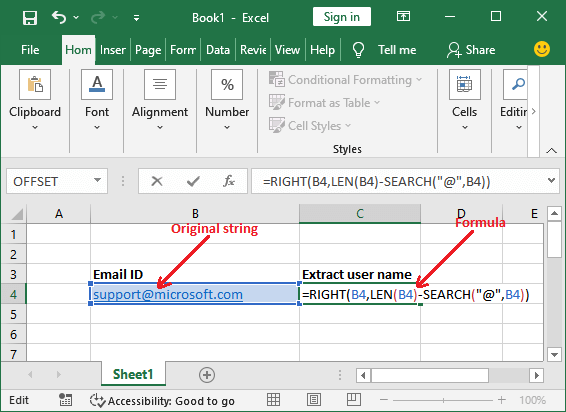Hit the Enter key and get the extracted domain name (substring before @ symbol) from the email id.### How this formula worked?

=RIGHT(B4, LEN(B4)-SEARCH(“@”, B4)) for the string [email protected].

The first thing to know that – this formula will execute from “right to left”. It needs maximum five statements to understand how this formula works. Read them carefully.

1. Firstly, the SEARCH() function will return the position of @ symbol in the original string (start counting from left and 1), i.e., 8.
2. Then, the LEN function will count the total length of the given string, i.e., 21.
3. Now, the position returned by the SEARCH function will be subtracted from the length of the string, i.e., 21-8 = 13.
4. This resultant value (13) will be used as the second parameter inside the RIGHT() function at the place of a number of characters to be extracted.
5. In the end, the RIGHT() function will extract the 13 rightmost characters from the string and return the substring, i.e., microsoft.com.

Next TopicCSV to Excel

Previous articleArrow key is not working in Excel
Next articleHow to share Excel sheet